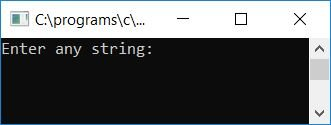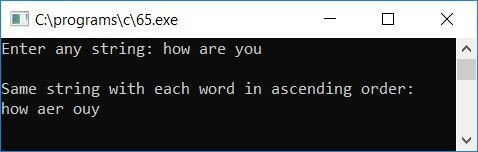# C program to sort each word from a given string in ascending order

In this article, we will learn about how to sort or arrange each word from any given string (by the user at run-time) in ascending order.

In this program, each word is sorted character-wise. For example, if the given string by the user is "this is codescracker," Here we have a total of three words ("this," "is," and "codescracker") present inside the string. Therefore, after sorting each word, the string will become "hist is acccdeekorrs." As you can see, all three words are sorted or arranged in ascending order.

```#include<stdio.h>
#include<conio.h>
int main()
{
char str, chTemp;
int i, j, len;
printf("Enter any string: ");
gets(str);
len = strlen(str);
for(i=0; i<len; i++)
{
for(j=0; j<(len-1); j++)
{
if(str[j]>=65 && str[j]<=90)
{
if(str[j+1]>=65 && str[j+1]<=90)
{
if(str[j]>str[j+1])
{
chTemp = str[j];
str[j] = str[j+1];
str[j+1] = chTemp;
}
}
}
if(str[j]>=97 && str[j]<=122)
{
if(str[j+1]>=97 && str[j+1]<=122)
{
if(str[j]>str[j+1])
{
chTemp = str[j];
str[j] = str[j+1];
str[j+1] = chTemp;
}
}
}
}
}
printf("\nSame string with each word in ascending order:\n%s", str);
getch();
return 0;
}```

The program was written in the Code::Blocks IDE; therefore, after a successful build and run, here is the sample run:Supply any string, say "how are you," and press the ENTER key to see the same string with its words sorted in ascending order as shown in the second snapshot of the sample run given here:Here is a list of some of the main steps used in the above program:

• Receive any string using the gets() function.
• Now find the length of the given string using the strlen() function of string. h library
• Store the length of a given string inside any variable, say len.
• Create a for loop that starts from 0 and ends at one less than the length of the string to compare each and every character.
• Inside the for loop, create another for loop to run or repeat comparing the string's every character.
• Inside the second for loop, confirm that both characters are alphabetic or not, and compare and reverse only when the previous character is greater than the next character.
• Let's suppose if the user supplies the string "codescracker," then c is compared with "o" first, as it is already arranged, then "o" is compared with the next character of the string, which is "d."
• Now we have to apply the reverse operation to place d before o (because o is greater than d) and continue to check for the next character, that is, o with e, and so on.
• In this way, all the characters of the string get sorted.
• We used two for looping to do comparing and reversing (if the previous character is greater than the next character) twice from start to end.
• Finally, print the value of the string on the output screen, which will be the same string but arranged in ascending order.

C Quiz

« Previous Program Next Program »